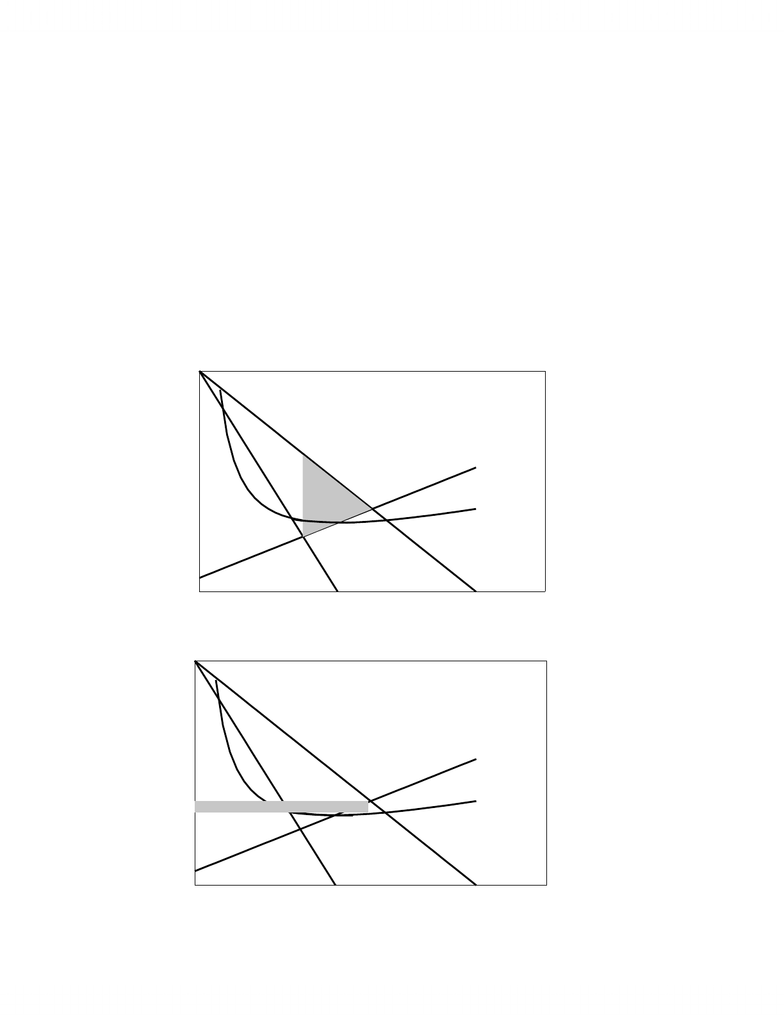# ECON 3K03 Lecture Notes - Lecture 11: Pareto Efficiency, Allocative Efficiency, Natural Monopoly

33 views8 pages
School
McMaster University
Department
Economics
Course
ECON 3K03
ProfessorPrinciples of Economics: Efficiency
EFFICIENCY
Definition: Productive Efficiency is optimal output from given resources or minimal cost for a
given output.
We have already seen the concept of productive efficiency in our discussion of Production
Possibilities.
Definition: Allocative Efficiency is the optimal allocation of resources
Allocative Efficiency implies not only the optimal output from given resources (Productive
Efficiency) but also the optimal output desired by society. Allocative Efficiency is the output
that maximizes the net social benefit of society, i.e., maximizes the difference between total
benefit and total cost to society.
Maximum Net Social Benefit => P = MC
Maximum Net Social Benefit implies the output where the benefit to society of an
additional unit of output equals the cost to society of an additional unit of output. It is the output
where Marginal Benefit equals Marginal Cost (MB = MC). Since we measure the marginal
benefit by willingness to pay, the market Demand for a commodity measures the Marginal
Benefit of a commodity. We can therefore say that the allocation of resources is optimal at the
output where Price equals Marginal Cost (P = MC).
Pareto Efficient Allocation (Pareto Optimality) => P = MC
Definition: Pareto Optimality is the allocation of resources where no person is worse off from
The Pareto Optimality condition is also satisfied by the output where P = MC.
- 1 -
Unlock document

This preview shows pages 1-3 of the document.
Unlock all 8 pages and 3 million more documents.Principles of Economics: Efficiency
Efficiency Loss (aka Welfare Loss or Deadweight Loss)
We measure the efficiency loss of an output Qo by the difference between Price and
Marginal Cost (Supply) between the output Qo and optimal output at P = MC. It is the
difference between Demand and Marginal Cost (Supply) between given output and optimal
output. We will see that this is equivalent to the net loss of consumers’ surplus and producers’
surplus at the output relative to optimal output.
Competition => Optimum Allocation (Allocation Efficiency, 0 Efficiency Loss)
Since firms and households are price-takers in a competitive market, competitive market
equilibrium implies that P = MC, which implies that competitive markets give the optimal
allocation of resources.
Monopoly Regulation
Monopolies create efficiency loss by reducing output below competitive output and
increasing price above competitive price. Governments cannot regulate monopolies through
taxation, though, since per unit taxes decrease monopoly output by shifting up Marginal Cost and
fixed taxes do not change monopoly output since marginal cost doesn’t change.
Governments attempt to eliminate the efficiency loss caused by a monopoly by requiring
that a ‘normal’ monopoly produce the output where Price equals Marginal Cost (P = MC) and
that a natural monopoly produce the output where Price equals Average Cost.
1. Marginal Cost Pricing
The principal concern of government regulation of monopoly is to eliminate efficiency loss
not to eliminate monopoly profits. Marginal Cost Pricing (P = MC) as the requirement that the
monopoly produce the output where Price equals Marginal Cost is therefore the preferred option
for government regulation. The upper diagram below shows the efficiency loss due to a normal
- 2 -
Unlock document

This preview shows pages 1-3 of the document.
Unlock all 8 pages and 3 million more documents.Principles of Economics: Efficiency
monopoly above and the lower diagram below shows the output, price, and economic profit and
loss of the monopoly given marginal cost pricing. You will note that marginal cost pricing
results in 0 efficiency loss but still leaves the monopoly with an economic profit. The monopoly
can still exist with greater than normal profits while society achieves optimal allocation of
resources. (This is only the short-run optimum allocation since firms would enter the competitive
market in the long-run so that 0 efficiency loss would occur where price equals marginal cost at
minimum average cost)
0 10 20 30 40 50
0
20
40
60
80 P, Cost/unit, MR
Q
Demand
MR
Qm
Pm MC
Efficiency
Loss
AC
P = 80 - 2Q; TC = Q
2
/2+5Q+200 => AC = Q/2 + 5 + 200/Q and MC = Q + 5
P = 80 - 2Q; TC = Q
2
/2+5Q+200 => AC = Q/2 + 5 + 200/Q and MC = Q + 5
0 10 20 30 40 50
0
20
40
60
80 P, Cost/unit, MR
Q
Demand
MR
Q
MC
P
MC
MC
AC
Economic Profit
- 3 -
Unlock document

This preview shows pages 1-3 of the document.
Unlock all 8 pages and 3 million more documents.

## Document Summary

Definition: productive efficiency is optimal output from given resources or minimal cost for a given output. We have already seen the concept of productive efficiency in our discussion of production. Definition: allocative efficiency is the optimal allocation of resources. Allocative efficiency implies not only the optimal output from given resources (productive. Efficiency) but also the optimal output desired by society. Allocative efficiency is the output that maximizes the net social benefit of society, i. e. , maximizes the difference between total benefit and total cost to society. Maximum net social benefit => p = mc. Maximum net social benefit implies the output where the benefit to society of an additional unit of output equals the cost to society of an additional unit of output. It is the output where marginal benefit equals marginal cost (mb = mc). Since we measure the marginal benefit by willingness to pay, the market demand for a commodity measures the marginal.